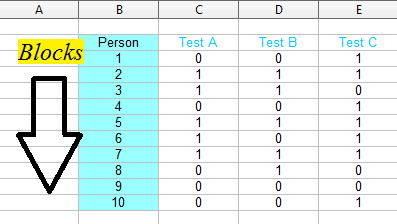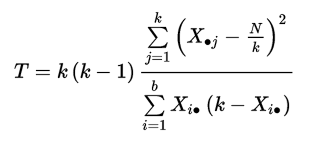# Cochran’s Q Test

Statistics Definitions > Cochran’s Q Test

## What is Cochran’s Q Test?

Cochran’s Q Test is a non parametric way to find differences in matched sets of three or more frequencies or proportions. It is an extension of the McNemar test; the two tests are equal if Cochran’s Q is calculated for two groups.

The test is usually used when you have a group of people performing a series of tasks or getting a set of treatments where the outcome is a “Success” or “Failure.” Like the binomial distribution, success/failure could mean yes/no, black/white or any one of a number of success/failure options.
Examples:

• Ten people perform 4 different logic problems. The tasks are the independent variable (with three groups) and the outcome of each task (pass or fail) is a dichotomous variable.
• Fifty people take three drugs, A, B, and C, to treat a disease. Outcomes are either favorable(success) or unfavorable(fail).

## Data Preparation

Most statistical software packages (e.g. SPSS) require that your data is entered in blocks. Usually a “block” is a person, like one person taking tests ABC. Enter a 1 for success and 0 for failure.### Null Hypothesis

The null hypothesis for Cochran’s Q is that the proportion of “successes” is equal for all groups. The alternate hypothesis is that the proportion is different for at least one group. The null hypothesis is rejected when the calculated Q critical value is greater than a critical chi-squared value.

Cochran’s will tell you if there is a difference, but it won’t tell you where those differences are. If you reject the null hypothesis (i.e. the test identifies differences), perform followup pairwise Cochran’s Q tests to identify the areas which have differences.

### Assumptions and Conditions

• You must have one dependent, dichotomous variable. Dichotomous variables are categorical variables with two categories or levels. The groups/levels must be mutually exclusive. For example, you could have “Pass” and “Fail”.
• You must have one independent variable with three groups or levels. For example, you might use three different tests or administer three different treatments.
• Ideally, use a random sampling method (e.g. simple random sampling).
• You must have a large enough sample size. A rule of thumb is that the number of subjects, n, multiplied by the number of levels in the independent variable, k, are greater than or equal to 24.

### Test Statistic

The test statistic for Cochran’s Q is calculated with the following test statistic:Where:

• b = the number of blocks,
• k = the number of treatments,
• X•j = column total for the jth treatment,
• Xi• = row total for the ith block,
• N = the grand total.

As you probably can tell just by looking at the formula, this can quickly get ugly with multiple summations. That’s why this test is nearly always performed with statistical software.

CITE THIS AS:
Stephanie Glen. "Cochran’s Q Test" From StatisticsHowTo.com: Elementary Statistics for the rest of us! https://www.statisticshowto.com/cochrans-q-test/